# Eqilibrium Constants For Different Types Of Equilibria

## Heterogeneous Equilibria

Heterogeneous equilibria are those which involve more than one phase among the reactants and products. For example, (i) Decomposition of solid CaCO3 into solid CaO and gaseous CO2. Let a moles of CaCO3 are taken in a vessel of volume V litre at temperature TK.

CaCO3(s)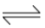CaO(s) + CO2(g)

Moles initially         a         0           0

Moles at equilibrium      a − x   x      x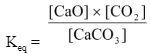Since, CaO and CaCO3 are pure solids, their concentrations do not change as it is given by d/M, so the given equilibrium expression can be rearranged as

Keq ×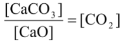It can be seen that left hand side of the equation is a constant represented by Kc.

∴Kc = [CO2] = x/v…(i)

Assuming CO2 gas to behave ideally, the molar concentration of CO2, [CO2] can be
written as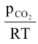.

∴Kc =Kc(RT) =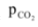Since, Kc, R and T are constants their product will also be a constant referred as Kp.

∴Kp =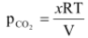…(ii)

From equation (i) and (ii), it is evident that whenever the equilibrium would be attained at TK, in a vessel of volume V litre, the moles of CO2 present at equilibrium must be x (which can exert a pressure equal to). If rather than starting with a mole of CaCO3, we start with x moles of CaCO3 in the vessel of volume V at T K, then the whole of CaCO3 would have decomposed to give CO2 but the equilibrium can not be maintained as there would be no CaCO3, further if the moles of CaCO3 taken in the same vessel at same temperature are less than x, the equilibrium would never to attained and any amount (moles) of CaCO3 more than x would be sufficient to establish the equilibrium. So the minimum moles of CaCO3 required to attain equilibrium under given conditions would be taken as x.

The given equilibrium can be made to propel in the forward direction by either removing some moles of CO2 or increasing the volume of the container or by increasing the temperature of the reaction (as the reaction is endothermic). Addition of solid CaCO3 or CaO to the equilibrium mixture would not affect the equilibrium situation.

For such equilibria, at any temperature, there will be a fixed value of the pressure of CO2, which is called as dissociation pressure. The variation of Kp with temperature is given by the relation.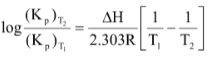### Simultaneous Equilibria

If more than one equilibria has been established in a vessel and any reactant(s) or product(s) is (or are) common to both the equilibria then the equilibrium concentration of the substance(s) in both the equilibria will be same. For example,

A + BC

At equi. a−x  a−x−y   x

B+ DE

At equi.     a−x−y    b−y   y

As reactant B is common to both the equilibrium, therefore the equilibrium concentration of B should be same for both the equilibria.

Example 4

### Equilibrium Constant for The Sum of Reactions

It is possible to determine the equilibrium constants for various reactions and then use them to obtain the equilibrium constant of other reactions. If a given chemical equation can be obtained by taking the sum of other equations, the equilibrium constant for the given equation equals the product of the equilibrium constants of the other equations. But remember that this is valid only when individual reactions are sequential i.e. one preceding other.